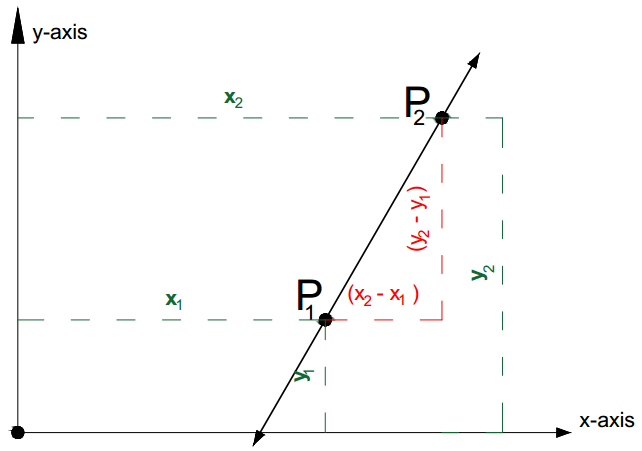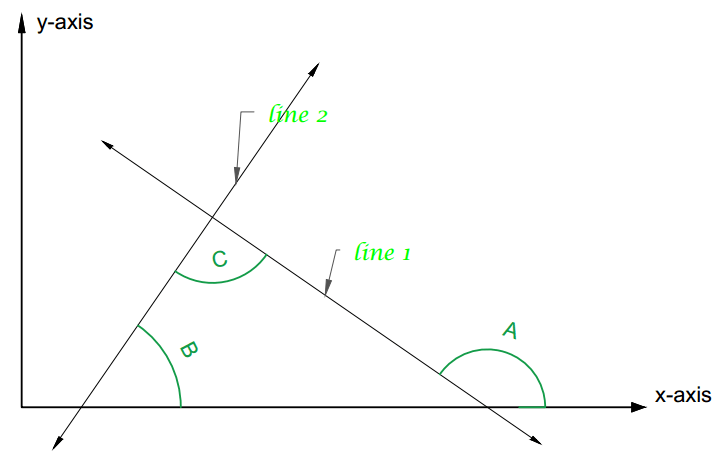### Math Notes

Subjects

#### Equation of a Line

SLOPE (m) of a line

$$m = \frac{rise}{run}=\frac{y}{x} = \frac{{{y_2} - {y_1}}}{{{x_2} - {x_1}}}$$Remarks:

Positive Slopes (m > 0). When x is increasing y is also increasing and vice versa. Typically described the line as sloping upwards to the right or downwards to the left.

Negative Slopes (m < 0). When x is increasing y is decreasing and when x is decreasing y is increasing. Characterized by a line moving downwards to the right or upwards to the left.

Zero Slopes (m = 0). These are lines parallel to x - axis.

Undefined Slopes (m = U). These are lines parallel to y - axis.

Parallel and Perpendicular lines

Slopes of Parallel Lines. m1 = m2. Two lines are parallel if they have the same slope and conversely.

Slopes of Perpendicular Lines. m1 = -1/m2. Two lines are perpendicular if and only if their slopes are negative reciprocals.

Given two lines 1 and 2. Assume that their slopes are negative reciprocal ($$m_1 = \frac{-1}{m_2}$$). Show that the angle of intersection is a right angle. This includes derivation on angles between lines.Assumption: $$m_1 = \frac{-1}{m_2}$$

Show that: $$\angle C = 90^o$$

Using the triangle formed. $$\angle B +\angle C = \angle A$$

$$\tan B = m_2$$ and

$$\tan A = m_1$$

$$\tan A = \tan (B+C)= m_1$$

$$\tan (B+C)= \frac{\tan B + \tan C }{1-\tan B \tan C} = \tan A$$

$$\tan A = \frac{m_2 + \tan C }{1- m_2 \tan C}$$

$$m_1= \frac{m_2 + \tan C }{1- m_2 \tan C}$$

$$\tan C = \frac{m_1-m_2}{1+m_2m_1}$$

But $$m_1 m_2 = -1$$ from the assumption

$$\tan C = \frac{m_1-m_2}{0} = U$$

Thus $$\angle C$$ must be a right angle.

Therefore, $$\angle C = 90^o$$

EQUATIONS OF A LINE

Let A, B, C, a, b are constants

1. General Form of a line.$$Ax + By + C = 0$$

2. Slope intercept form of a line $$y = mx + b$$

3. Point - Slope form of a line $$(y - {y_1}) = m(x - {x_2})$$

4. Two point form $$\frac{{{y_2} - {y_1}}}{{{x_2} - {x_1}}} = \frac{{y - {y_1}}}{{x - {x_1}}}$$

5. Intercept form. $$\frac{x}{a} + \frac{y}{b} = 1$$

a = x - intercept. The value of x when y is zero.

b = y - intercept. The value of y when x is zero.